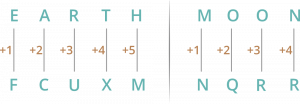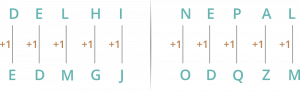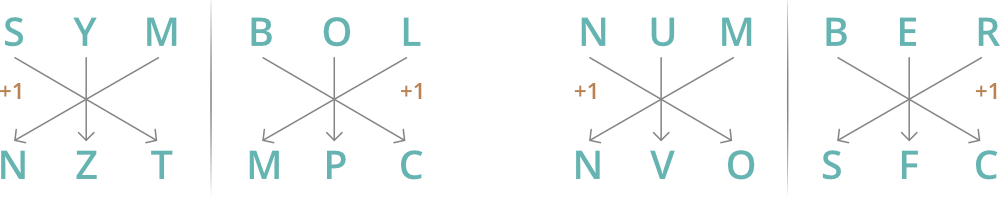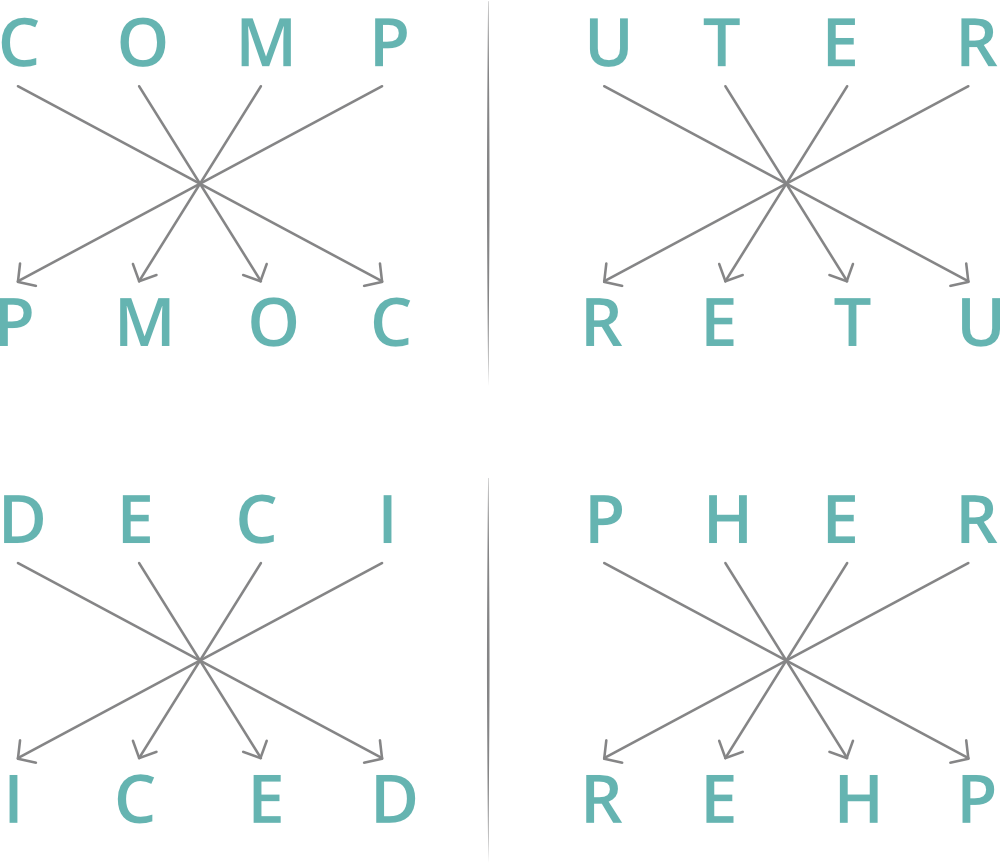# Coding Decoding Question and Answers

Coding decoding is an important part of the Quantitative Aptitude section in most of the competitive examinations in India. Coding and decoding questions are a common feature in the reasoning ability section of major Government exams such as those conducted by banks, insurance companies, SSC, and RRB. These types of questions test a candidate’s ability to decode a given condition or code presented in the statement and then answer the related questions based on it.

### Coding-Decoding Reasoning

Coding decoding is a reasoning skill that is frequently tested in various competitive government exams. Although this section might seem tricky at first, it is essential to attempt these questions as they often carry easy marks. In each question, you will be given a word and its coded form, and you are required to decode the logic behind the coding. Once you have figured out the logic, you can then apply it to another given word to find its coded form. With a little bit of practice, anyone can become proficient in this skill!

Tricks to Solve Coding Decoding Problems

## Types of Coding Decoding

### 1. Letter Coding

Letter coding is a method of changing information from its original form into coded messages. It involves things like converting words or phrases into other words or symbols using specific patterns or rules. Essentially, the message is encrypted so that only those who possess the key can read and understand it.

### 2. Number Coding

Number coding is a method of assigning specific numerical values to words, phrases, or numbers that can later be decrypted using predefined rules. This technique is commonly used in computer programming and data security and also in covert communication.

### 3. Substitution Coding

It is a type of puzzle-solving that involves substituting specific words for other names.

## Sample Questions on Coding Decoding

### Q1. If EARTH is written as FCUXM in a certain code. How is MOON written in that code?

Solution### Q2. If DELHI is written as EDMGJ in a certain code. How is NEPAL written in that code?

Solution :### Q3. If SYMBOL is written as NZTMPC is it a certain code? How is NUMBER written in that code?

Solution

Acc. to question### Q4. In a certain code, COMPUTER is written as PMOCRETU, how is DECIPHER written in that code?

Solution

Acc. to question### Q5. In a certain code, NEWYORK is written as 111, how is NEWJERSEY written in that code?

Solution

In NEWYORK
N = 14, E = 5, W = 23, Y = 25, O = 15, R = 18, K = 11
Total = 14 + 5 + 23 + 25 + 15 + 18 + 11 = 111
In NEWJERSEY
Total = 14 + 5 + 23 + 10 + 5 + 18 + 19 + 5 + 25
= 124

### Q6. In a certain code, HARYANA is written as 8197151, how is DELHI written in that code?

Solution

We used the number of alphabets here.
H = 8
A = 1
R = 18 = 1+8 = 9
Y = 25 = 2+5 = 7
For DELHI
D = 4
E = 5
L = 12 = 1+2 = 3
H = 8
I = 9
Hence, DELHI is written as 45389.

### Q7. In a certain code BOMB is written as 5745 and BAY is written as 529, how is BOMBAY written in that code?

Solution

Acc. to question
Use the numbers to relate the words

```      B O M B      B A Y      ->          B O M B A Y
5 7 4 5      5 2 9                  5 7 4 5 2 9```

## Practice Problems on Coding Decoding:

### Q1. If in a certain language, MADRAS is coded as NBESBT, how is BOMBAY coded in that code?

(a) CPNCBX (6) CPNCBZ (c) CPOCBZ (d) CQOCBZ (e) None of these

### Q2. In a certain code, TRIPPLE is written as SQHOOKD. How is DISPOSE written in that code?

(a) CHRONRD (6) DSOESPI (c) ESJTPTF (d) ESOPSID (e) None of these

### Q3. If in a code language. COULD is written as BNTKC and MARGIN is written as LZQFHM, how will MOULDING be written in that code?

(a) CHMFINTK (6) LNKTCHMF (c) LNTKCHMF (d) NITKHCMF (e) None of these

### Q4. In a certain code, MONKEY is written as XDJMNL. How is TIGER written in that code?

(a) QDFHS (6) SDFHS (c) SHFDQ (d) UJHFS (e) None of these

### Q5. If FRAGRANCE is written as SBHSBODFG, how can IMPOSING be written?

(a) NQPTJHOJ (6) NQPTJOHI (c) NQTPJOHJ (d) NQPTJOHJ (c) None of these